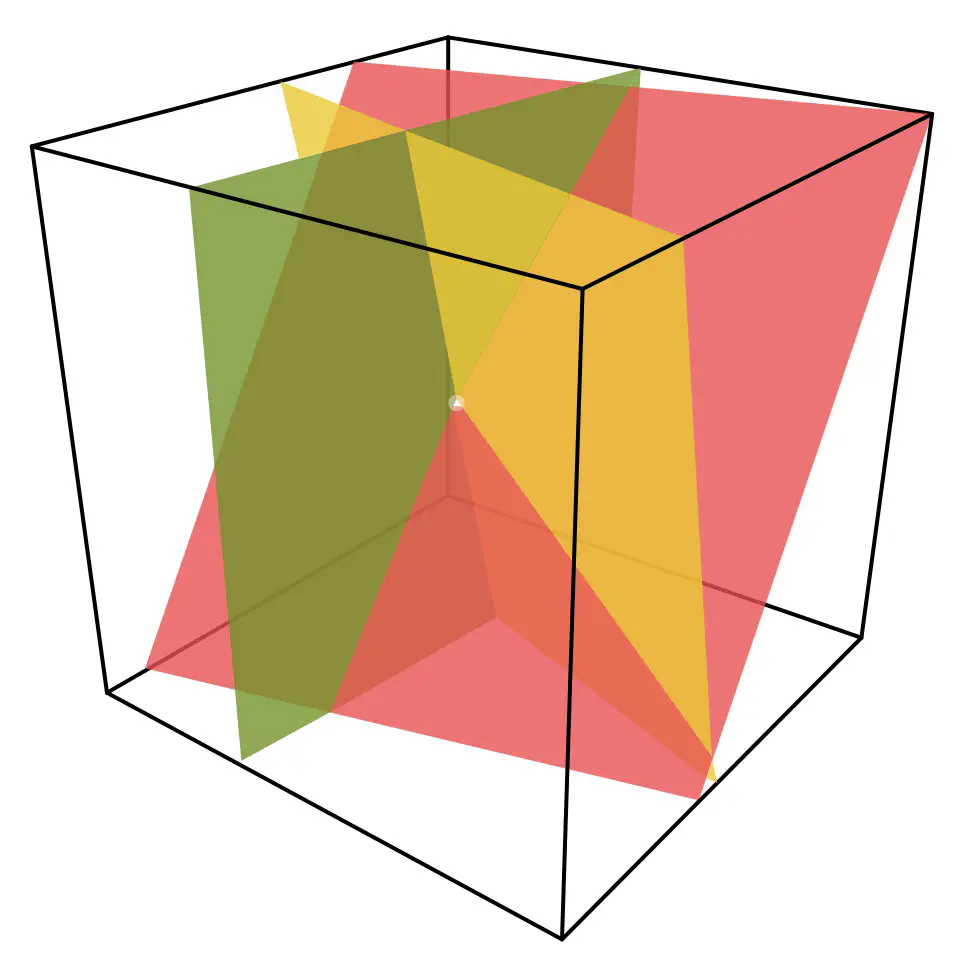# Linear Algebra

Umeå University course 5MA160.Image credit: from Wikipedia

This is the basic course 5MA160 in linear algebra aimed at first year students. We cover the usual elements of most linear algebra courses including solving systems of linear equations, manipulating matrices, computing eigenvalues, and working with the fundamental theorem of linear algebra. One goal of the course is to build geometric intuition in the students by studying vectors in 2 and 3 dimensional spaces so that they might better understand higher dimensional spaces. The course covers both abstract concepts such as the theory of general vector spaces as well as practical applications such as markov chains and networks. Students are evaluated by exams and a computer lab that requires the students to use MATLAB to apply their linear algebra knowledge.

The primary text is Elementary linear algebra: with supplemental applications 12th edition by Anton Howard and Rorres Chris (ISBN 9781119666141). Additional material is drawn from Introduction to Linear Algebra 4th edition by Gilbert Strang, Linear Algebra 3rd edition by Jim Hefferon, and videos by 3blue1brown on the Essence of linear algebra##### Eric Libby
###### Associate professor

My research addresses how simple organisms evolve to be complex.